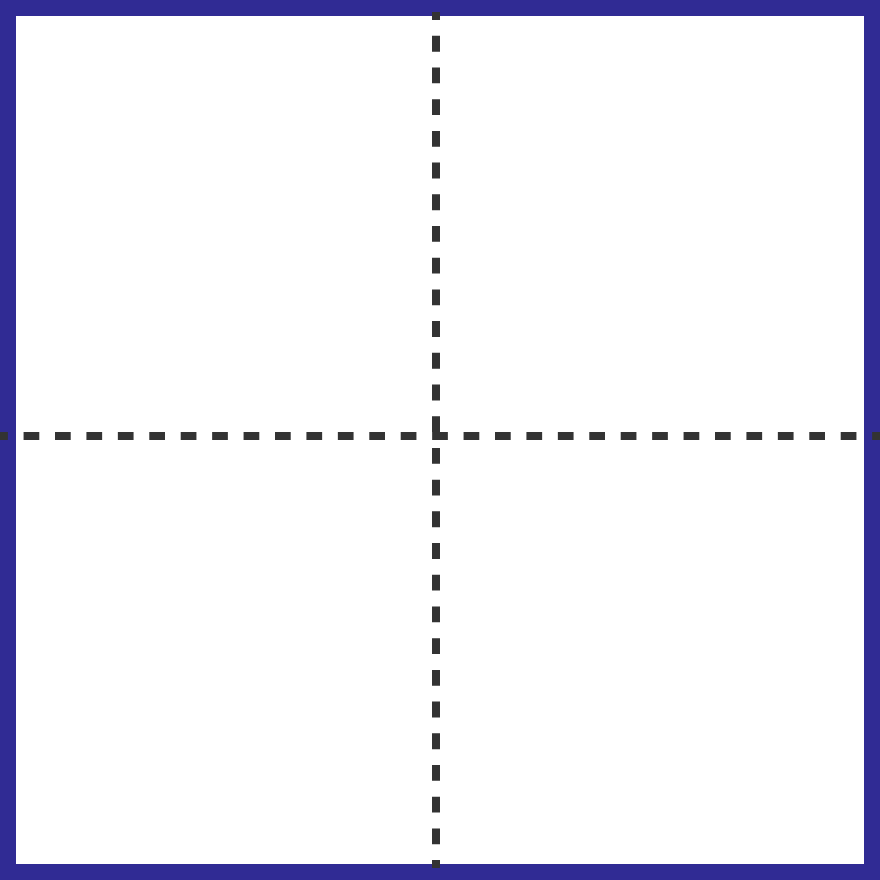# Cutting a square

Geometry Level 3

I've partitioned a unit square into 4 rectangles and each rectangle has perimeter 2, as illustrated in the diagram. I can also cut this unit square into all of the following numbers of rectangles, each with perimeter 2, except for one number.

Which one is it?×